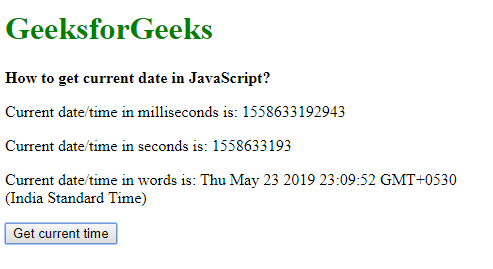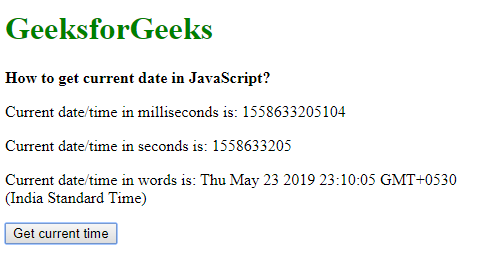# How to get the current date and time in seconds?

Method 1: Using Date.now() method: The Date.now() method returns the number of milliseconds elapsed since January 1, 1970 00:00:00 UTC. This is known as the epoch time. It may be used for timestamping as an order of events could easily be checked by comparing the timestamps.

The returned milliseconds can be converted to seconds by dividing the value by 1000 and then using the Math.round() function to round the value. This is done to prevent inconsistencies due to the float values.

Syntax:

`Date.now()`

Example:

 ` ` `<``html``> ` `     `  `<``head``> ` `    ``<``title``> ` `        ``How to get current date in JavaScript? ` `    `` ` ` ` ` `  `<``body``> ` `    ``<``h1` `style``=``"color: green"``> ` `        ``GeeksforGeeks ` `    `` ` `     `  `    ``<``b``> ` `        ``How to get current date in JavaScript? ` `    `` ` `     `  `    ``<``p``> ` `        ``Current date/time in milliseconds is:  ` `        ``<``span` `class``=``"output-msecs"``> ` `    `` ` `     `  `    ``<``p``> ` `        ``Current date/time in seconds is:  ` `        ``<``span` `class``=``"output-secs"``> ` `    `` ` `     `  `    ``<``p``> ` `        ``Current date/time in words is:  ` `        ``<``span` `class``=``"output-words"``> ` `    `` ` ` `  `    ``<``button` `onclick``=``"getCurrentDate()"``> ` `        ``Get current time ` `    `` ` `     `  `    ``<``script` `type``=``"text/javascript"``> ` `     `  `        ``function getCurrentDate() { ` `            ``dateInMillisecs = Date.now(); ` ` `  `            ``// rounding the value to prevent inconsistencies ` `            ``// due to floating points ` `            ``dateInSecs = Math.round(dateInMillisecs / 1000); ` ` `  `            ``dateInWords = new Date(dateInMillisecs); ` ` `  `            ``document.querySelector('.output-msecs').textContent ` `                    ``= dateInMillisecs; ` `                     `  `            ``document.querySelector('.output-secs').textContent ` `                    ``= dateInSecs; ` `                     `  `            ``document.querySelector('.output-words').textContent ` `                    ``= dateInWords; ` `        ``} ` `    `` ` ` ` ` `  `                     `

Output:Method 2: Using new Date.getTime() method: The Date.getTime() method returns the number of milliseconds elapsed since January 1, 1970 00:00:00 UTC. It always uses the UTC for time representation. It has to be initialized with the new keyword, unlike the Date.now() method.

The returned milliseconds can be converted to seconds by dividing the value by 1000 and then using the Math.round() function to round the value. This is done to prevent inconsistencies due to the float values.

Syntax:

`new Date().getTime()`

Example:

 ` ` `<``html``> ` `     `  `<``head``> ` `    ``<``title``> ` `        ``How to get current date in JavaScript? ` `    `` ` ` ` ` `  `<``body``> ` `    ``<``h1` `style``=``"color: green"``> ` `        ``GeeksforGeeks ` `    `` ` `     `  `    ``<``b``> ` `        ``How to get current date in JavaScript? ` `    `` ` `     `  `    ``<``p``> ` `        ``Current date/time in milliseconds is:  ` `        ``<``span` `class``=``"output-msecs"``> ` `    `` ` `     `  `    ``<``p``> ` `        ``Current date/time in seconds is:  ` `        ``<``span` `class``=``"output-secs"``> ` `    `` ` `     `  `    ``<``p``> ` `        ``Current date/time in words is:  ` `        ``<``span` `class``=``"output-words"``> ` `    `` ` ` `  `    ``<``button` `onclick``=``"getCurrentDate()"``> ` `        ``Get current time ` `    `` ` `     `  `    ``<``script` `type``=``"text/javascript"``> ` `     `  `        ``function getCurrentDate() { ` `            ``dateInMillisecs = new Date().getTime(); ` ` `  `            ``// Rounding the value to prevent inconsistencies ` `            ``// due to floating points ` `            ``dateInSecs = Math.round(dateInMillisecs / 1000); ` ` `  `            ``dateInWords = new Date(dateInMillisecs); ` ` `  `            ``document.querySelector('.output-msecs').textContent ` `                    ``= dateInMillisecs; ` `                     `  `            ``document.querySelector('.output-secs').textContent ` `                    ``= dateInSecs; ` `                     `  `            ``document.querySelector('.output-words').textContent ` `                    ``= dateInWords; ` `        ``} ` `    `` ` ` ` ` `  `                     `

Output:My Personal Notes arrow_drop_upIm listening

If you like GeeksforGeeks and would like to contribute, you can also write an article using contribute.geeksforgeeks.org or mail your article to contribute@geeksforgeeks.org. See your article appearing on the GeeksforGeeks main page and help other Geeks.

Please Improve this article if you find anything incorrect by clicking on the "Improve Article" button below.